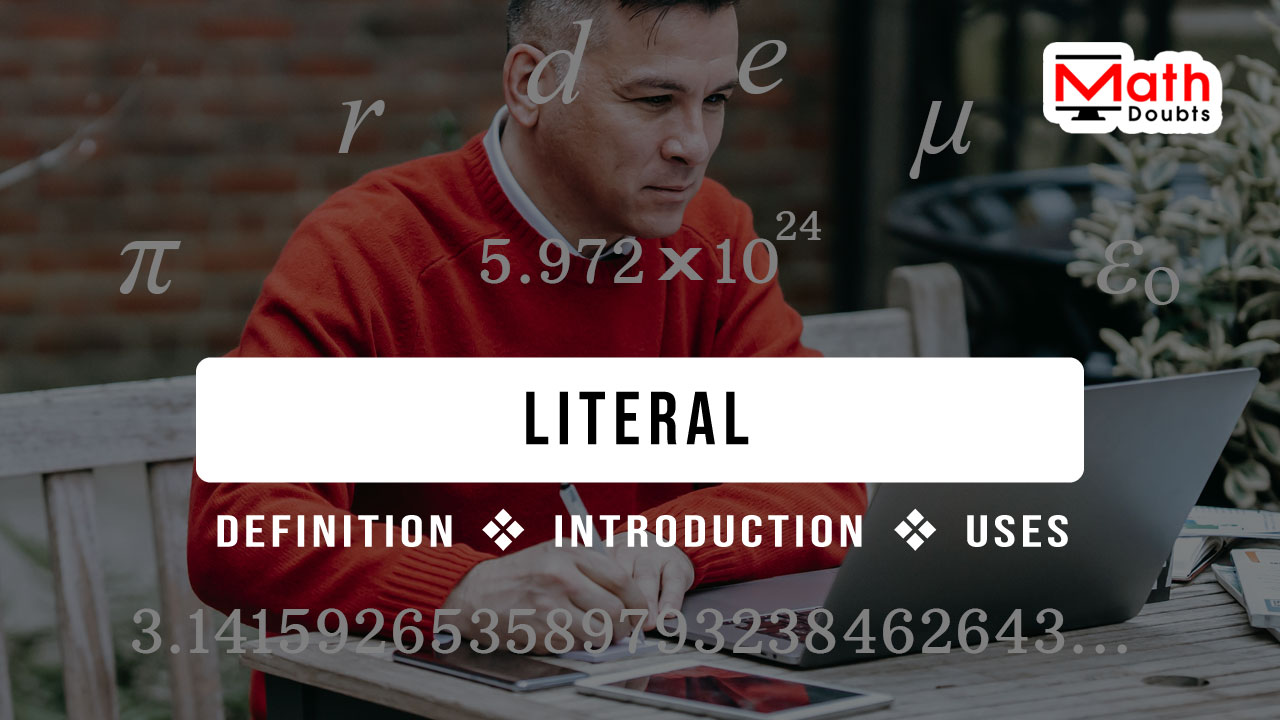# What is a Literal?

A letter that represents a quantity is called a literal.

## Introduction

The literal is a first and foremost mathematical concept to start learning the algebra. So, it is essential for those who learn the algebra newly should understand what exactly a literal is!In arithmetic, the quantities are expressed in the numerals. The same concept was used to introduce the algebra by expressing the quantities in the letters. Remember that you can use letters of any language.

According to the English language, the word ‘literal’ means expressed by a letter. Hence, a letter that expresses a quantity is called a literal. Similarly, it is also called a literal number.

### Example

The number of alphabets in English language is twenty six. According to the arithmetic, it is expressed by a numeral $26$ in mathematics.

In algebra, it is denoted by a letter and you can represent it by any letter of any language. Let us represent the number of alphabets by $A$ subscript $e$.

$A_e} \,=\, 2$

Now, we should recognize $A$ subscript $e$ as the number of alphabets in English language and it is equal to $26$. The $A$ subscript $e$ represents a quantity. So, it is called a literal or literal number in algebra.

### Essentiality

Any quantity can be expressed in real numbers. A doubt may raise in you that then what is the necessity of introducing algebra in mathematics?

It is obviously a genuine question but the following two irrational numbers clarify you about the importance of algebra in mathematics and also importance of literals in algebra.

$(1).\,\,$ $2.7182818284\cdots$

$(2).\,\,$ $3.1415926535\cdots$

The two numbers are scientifically very important in mathematics, physics and engineering but it is too hard to remember and also too tough to write them as many times as we want. So, it is recommended to represent them by literals to overcome the problems in both remembering and writing them.

The first number is denoted by a letter $e$, which is called Euler’s number and the second number is represented by a letter pi ($\pi$).

$(1).\,\,$ $e$ $\,=\,$ $2.7182818284\cdots$

$(2).\,\,$ $\pi$ $\,=\,$ $3.1415926535\cdots$

The letters $e$ and $\pi$ represent two quantities. Therefore, they are called literals as per the algebraic mathematics. Now, the two irrational numbers can be easily remembered and can also be written easily as many times as we want in terms of both literal numbers.

Latest Math Topics
Jun 26, 2023
Jun 23, 2023

###### Math Questions

The math problems with solutions to learn how to solve a problem.

Learn solutions

Practice now

###### Math Videos

The math videos tutorials with visual graphics to learn every concept.

Watch now

###### Subscribe us

Get the latest math updates from the Math Doubts by subscribing us.